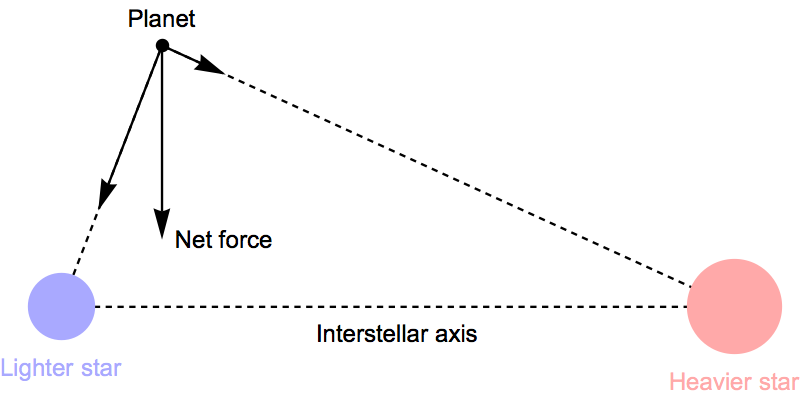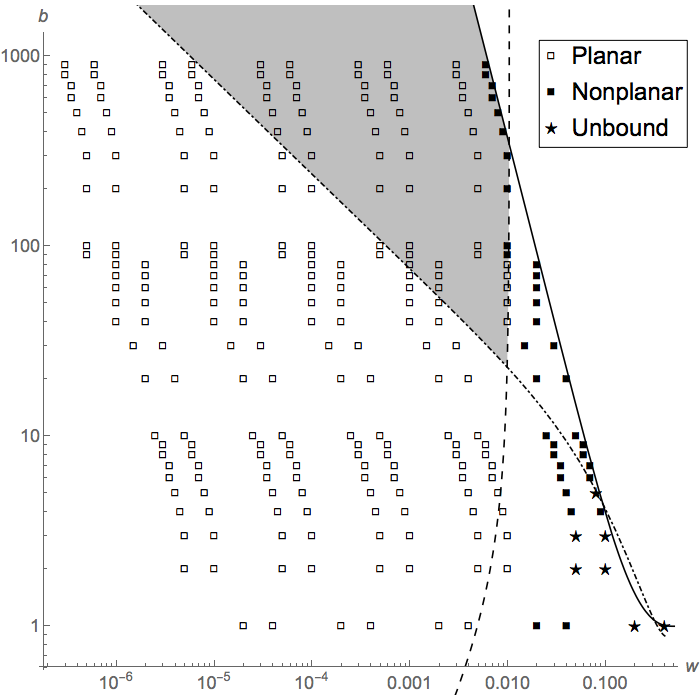# Polar Orbits Around Binary Stars

## by Greg Egan

This web page shows the results of some numerical simulations for a paper I wrote, “Polar Orbits Around Binary Stars”.

Suppose two stars in a binary system orbit their common centre of mass in perfectly circular orbits. We wish to explore the behaviour of a planet, of negligible mass compared to the stars, that initially moves in a circular orbit that is orthogonal to the axis between the two stars.

To determine the initial position and velocity of the planet, we choose a parameter w, which describes how far along the axis from the lighter star towards the heavier the plane of the orbit is situated, with w=0 for an orbit centred on the lighter star, and w=1 for an orbit centred on the heavier star. We will also choose a parameter b that gives the ratio of the stars’ masses.We then compute the distance from the interstellar axis such that the axial components of the forces on the planet from the two stars cancel each other out exactly, leaving a net force on the planet directed entirely towards the axis, as in the figure on the left. (There will be a range of values for w where this is impossible. For example, if the stars have unequal masses, and w=0.5, then whatever distance the planet lies from the axis, its distance from both stars will be the same, and the angle their force vectors make with the axis will be the same, so the heavier star will always pull the planet towards it along the axis more strongly.)

If the two stars of the binary pair were kept motionless, then for values of w where we can balance the gravitational forces along the axis, we could put a planet into a circular orbit around the axis, with the net gravitational force towards the axis providing the necessary centripetal force. Some of these orbits will be stable under small perturbations to the planet’s position and velocity, but some will be unstable; we will only use choices of w and b where these orbits are stable.

If the stars remained motionless, then the planet would simply execute a stable circular orbit like this forever. But we wish to study what happens when the stars are instead allowed to proceed in their own orbit. So we start the planet’s orbit in the same position relative to the two stars (specifically, at one of the points in the plane of the stellar orbit where the planetary orbit crosses that plane), and give it an initial velocity whose component orthogonal to the plane is the same as it would have if it were executing a circular orbit around the axis with the stars held motionless. But we also give its initial velocity a small component within the plane of the stellar orbit, such that it is neither moving away from, nor towards, either of the stars, despite the fact that they are both now in motion. To put it another way: to an observer using a reference frame that rotates with the stars, so that they appear to be motionless, the planet is initially moving relative to the stars in exactly the same way as it was when they were motionless.Sample points in the parameter space close to the lighter star.Sample points in the parameter space close to the heavier star.

But of course, we don’t expect that to continue to hold true: once the stars are allowed to move in their own orbit, there is no reason why the planet should continue to maintain the relationship with them that held when they were motionless.

The motion of a planet starting from these initial conditions was integrated numerically for 540 choices of the parameters w and b. The values of these parameters are plotted in the figures on the right. Here, the unbroken lines mark the boundaries of the regions where the circular orbits around the axis when the stars are held fixed are stable, while the dashed lines mark the boundaries of the regions where the frequency of the planetary orbit is at least ten times faster than the stellar orbit (with the planetary orbit being smaller and faster the closer it lies to one of the stars).

[In the upper figure, the dot-dashed line delimits the region where (according to an analysis by Oks , after correcting for algebraic errors in both these papers) the radial perturbations induced by the motion of the stars in the original circular orbits are less than half the radius of the orbit itself. There are 59 points (those lying in the shaded region of the top figure) for which all the stated prerequisites for the analysis by Oks are satisfied. However, the analysis itself is invalid, as the results below demonstrate.]

For each choice of parameters, the planet’s trajectory was computed for one complete stellar orbit. The integration was performed in Mathematica, using 200 digits of working precision. As a check on the results, the Jacobi integral (a quantity that is conserved in the Circular Restricted Three-Body Problem) was computed at 10,000 time points for each trajectory. The greatest proportional deviation from the original value, for all time points and all trajectories, was 2.56 × 10–8, while the median value, for all 540 trajectories, of the maximum proportional deviation during the trajectory, was 7.25 × 10–16.

The results were classified into three broad categories. If, after one stellar orbit, the kinetic energy of the planet exceeded its potential energy in relation to the star to which it was originally bound, the orbit was classified as unbound. Otherwise, if the displacement vector to the planet from that star never deviated by more than 15° on either side of the original plane of the orbit, the orbit was classified as planar. Essentially, such orbits are ordinary polar orbits around one of the stars of the binary pair, albeit perturbed to some degree by the companion star. In other cases with more complex trajectories, the orbits were classified as nonplanar.

Note that these calculations do not test the long-term stability of any of the orbits. This issue is discussed by Innanen et al. .

From the figures on the right, it is apparent that those planetary orbits that start out very close to one of the stars remain planar on the time scale of a single stellar orbit. As the parameters approach the curve that marks the transition between stable and unstable orbits (when the stars are held motionless), the planetary orbits shift to nonplanar, and then in some cases they become unbound within a single stellar orbit.

Plots of the planet’s trajectory in all 540 cases can be viewed by clicking on the controls below.Near lighter star Near heavier star Mass ratio 1 Frame 1 of 10

After the parameter map on the left, the first image is a plot of the planet’s trajectory, in a non-rotating frame centred on the star to which it is initially closest. If the trajectory is so large that showing it brings the other star into view, it is drawn as a ring of spheres, since in the course of one stellar orbit it completes a circle around its companion.

The image on the right is a plot of M(t), the component of the orbital angular momentum of the planet along the interstellar axis, computed in a rotating frame locked to the motion of the stars. The plots are normalised by dividing M(t) by its initial value at time zero, and the time along the horizontal axis is given as a fraction of the period of the stellar orbit, τS. The purpose of these angular momentum plots is to refute the assumption in Oks  that M(t) will remain approximately constant throughout the stellar orbit. This is clearly not true for any of the sample points, including the 59 points that meet all the stated prerequisites for the analysis in that paper.

## References

 “Polar Orbits Around Binary Stars” by Greg Egan, Celestial Mechanics and Dynamical Astronomy, (2018) 130:5. Online at Springer Web Site.

 “Stable Conic-Helical Orbits of Planets Around Binary Stars: Analytical Results” by E. Oks, The Astrophysical Journal, 804:106 (11pp), 2015 May 10. Online at IOP Web Site.

 “Erratum: Stable Conic-Helical Orbits of Planets Around Binary Stars: Analytical Results” by E. Oks, The Astrophysical Journal, 823:69 (1pp), 2016 May 20. Online at IOP Web Site.

 “The Kozai Mechanism and the Stability of Planetary Orbits in Binary Star Systems” by K.A. Innanen, J.Q. Zheng, S. Mikkola & M.J. Valtonen, The Astronomical Journal, Volume 113, No. 5, pp 1915–1919, May 1997. Full article available at Smithsonian Astrophysical Observatory/NASA Astrophysics Data System.Science Notes / Polar Orbits Around Binary Stars / created Sunday, 30 July 2017